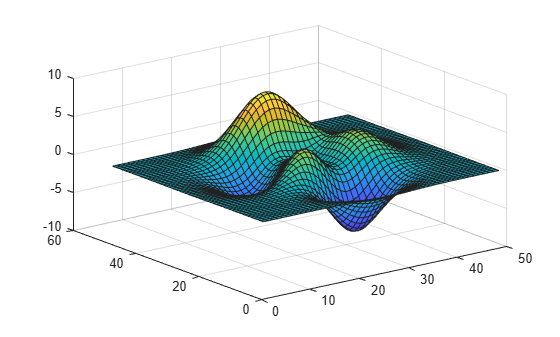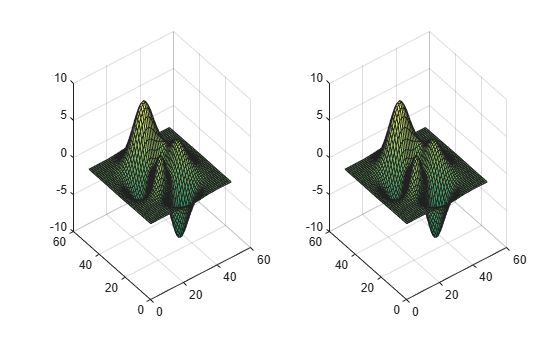# brighten

## 语法

``brighten(beta)``
``brighten(map,beta)``
``newmap = brighten(___)``
``brighten(f,beta)``

## 说明

``brighten(beta)` 沿同一方向变换当前颜色图中所有颜色的强度。当 `beta` 介于 `0` 和 `1` 之间时，颜色变亮；当 `beta` 介于 `-1` 和 `0` 之间时，颜色变暗。变化的幅度与 `beta` 的幅值成正比。使用此语法，可以调整当前图窗中使用颜色图的所有图形对象的颜色。`

``brighten(map,beta)` 变换指定为 `map` 的颜色图的强度。`
``newmap = brighten(___)` 为上述语法中的任意输入参数组合返回调整后的颜色图。指定输出参数时，当前图窗不受影响。`
``brighten(f,beta)` 变换为图窗 `f` 指定的颜色图的强度。其他图形对象（例如坐标区、坐标区标签和刻度）的颜色也会受到影响。`

## 示例

`surf(peaks);``brighten(.8);````t = tiledlayout(1,2); ax1 = nexttile; surf(ax1,peaks); ax2 = nexttile; surf(ax2,peaks); colormap(summer)``````newmap = brighten(summer,-.7); colormap(ax2,newmap)```## 输入参数

`$\gamma =\left\{\begin{array}{cc}1-\beta ,& \beta >0\\ \frac{1}{1+\beta },& \beta \le 0\end{array}$`

```map = [0.2 0.1 0.5 0.1 0.5 0.8 0.2 0.7 0.6 0.8 0.7 0.3 0.9 1 0];```

`brighten(parula,.8)`